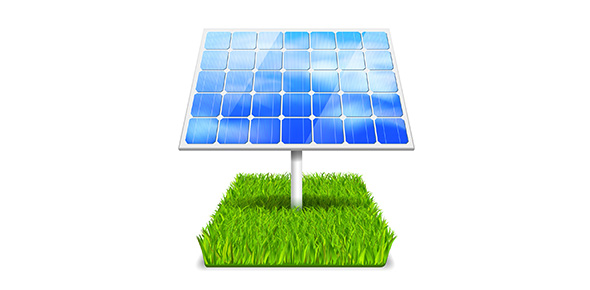# Energy Transformation Trivia: MCQ Quiz!

23 Questions | Attempts: 299
ShareSettingsCan you explain energy transformation? Energy transformation is also identified as energy conversion. It is the process of shifting energy to one form or another. The energy in several of its forms may be utilized in natural processes or to offer something to people, such as heating, refrigeration, lighting, or mechanical work to operate machines. Remember to obtain your certificate once this quiz is over.

• 1.
Energy is defined as?
• A.

The capacity to do work

• B.

The capacity to produce heat

• C.

The capacity to cause change

• D.

All of these

• 2.
Energy can be ________________ from one form to another.
• 3.
The SI unit for energy is the ____________ .
• 4.
James Joule deomonstrated that ___________ can be convereted into _________ .
• 5.
There are many forms of energy, each of which can be classified into one of three major categories.  Which three?
• A.

• B.

Kinetic

• C.

Potential

• D.

Emotion

• 6.
Lifting a medium-sized potato a distance of 1 m would require approximately _________ joule of energy.
• 7.
Another unit for energy, which is commonly used to quantify the energy in food is the _________ .
• 8.
One calorie is the amount of heat needed to raise the __________ of one gram of water by one degree Celsius.
• 9.
One calorie is equal to ________ joules.
• A.

4.184

• B.

4184

• C.

100

• D.

1/4

• 10.
Kinetic energy is the energy of an object in _________ .
• 11.
The kinetic energy of an object can cause another object to do _________ by colliding and causing it to move a particular ___________ .
• 12.
Which of these are examples of colliding objects?
• A.

A hammer swinging down on a nail

• B.

Two atoms colliding in a chemical reaction

• C.

A soccer player kicking a ball

• D.

A book sitting on a shelf

• 13.
One example of kinetic energy is ______________ energy which is caused by the motion of parts.
• 14.
Another example of kinetic energy is ______________ energy which is caused by the random motion of particles of matter.
• 15.
An object that has ____________ is not moving or doing work.
• 16.
If an object has potential energy, it means that at some point in the past, that object had work performed on it that resulted in energy ____________ .
• 17.
One example of performing work on an object to give it potential energy is __________ a watch.  As it is being tightened, the spring is gaining potential energy.
• 18.
Winding a watch transofrms _______________ energy to __________ energy.
• 19.
Another example of potential energy is water in an elevated tank.  If water is allowed to fall on a wheel and the wheel turns, the weel can be used to produce electricity.  The ___________ energy of the water in the tank is transformed into the ___________ energy of the wheel, which was further transformed into ______________ energy.
• 20.
Conversion between potential energy and kinetic energy is quite common.  As the potential energy of an object increases, its kinetic energy tends to ____________ .
• 21.
If the kinetic energy of an object increases, its potential energy __________ .
• 22.
A rubber ball held out of a third-story window has potential energy.  If it is released, gravity causes it to fall.  As it falls, it ______________ potential energy because its height decreases.  It ___________ kinetic energy because its velocity is increasing.
• 23.
A ball is dropped from a third-story window.  When the ball reaches the ground, it has lost all of its ____________ energy (because it cannot fall any further) and at the same time reached a _____________ kinetic energy.

## Related TopicsBack to top
×

Wait!
Here's an interesting quiz for you.